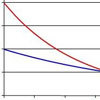#### You may also like### PDF

Given a probability density function find the mean, median and mode of the distribution.### Scale Invariance

By exploring the concept of scale invariance, find the probability that a random piece of real data begins with a 1.### Into the Exponential Distribution

Get into the exponential distribution through an exploration of its pdf.

# PCDF

### Why do this problem?

This problem will require learners to engage with the key properties of pdfs and cdfs and to understand how these relate to actual functions. The reasoning required is quite sophisticated, although the actual answer might be relatively short.

The problem would be of particular value either at the start or the end of a body of work on pdfs and cdfs.

### Possible approach

The first obstacle to overcome is to understand properly the problem, as it might be more formally stated than students are used to. Once this is done, students should be encouraged to think about the properties of pdfs and cdfs, and then to start addressing the problem. As there is little 'calculation' required, encouragement and discussion will most likely be of use.

Part of the problem is showing or explaining why it is that certain forms which would work as a cdf cannot be used as pdf, or vice-versa, so the emphasis should be on clear reasoning.

It is important to be aware the the problem does not require any assessment of the type of probability process underlying the proposed pdfs and cdfs: it can, and should, be done entirely algebraically.

### Key questions

What is the single most important constraint that a function being used as a pdf must satisfy?
What are the constraints on a function which is to be used for a pdf?

### Possible extension

Can you describe a probability process which would give rise to the pdfs and cdfs you come up with in the problem?

### Possible support

Look at some concrete examples of pdfs and cdfs. In each specific case, what is it that prevents the pdf being used as the cdf, or vice versa?